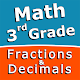Home » Education » Third grade Math skills - Fractions and Decimals# Third grade Math skills - Fractions and Decimals

Sergey Malugin

(1)
Test, practice and improve you math skills. Includes three fun mini games.

## The description of Third grade Math skills - Fractions and Decimals

Intuitive interface powered by handwriting input and a choice of three fun and engaging mini games in addition to a regular math trainer mode make our app stand out from the crowd of generic math learning apps.

With Third grade Math skills - Fractions and Decimals you can practice and improve the following math skills:
- Add fractions with like denominators
- Subtract fractions with like denominators
- Write fractions in lowest terms
- Subtract decimal numbers
- Convert decimals to fractions and mixed numbers
- Convert fractions and mixed numbers to decimals

Choose between four different modes:
- Math Trainer
- Math Shot mini-game
- Math Gravity mini-game
- Math Trick Shot mini-game
Category: Free Education App
Publish Date: December 09, 2018